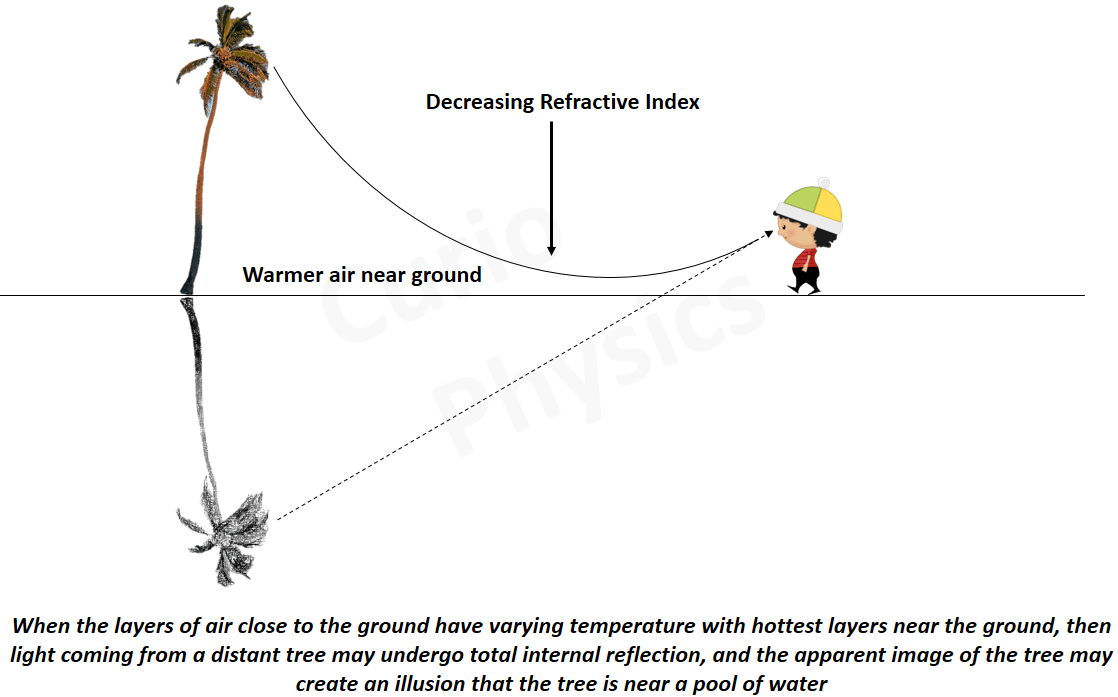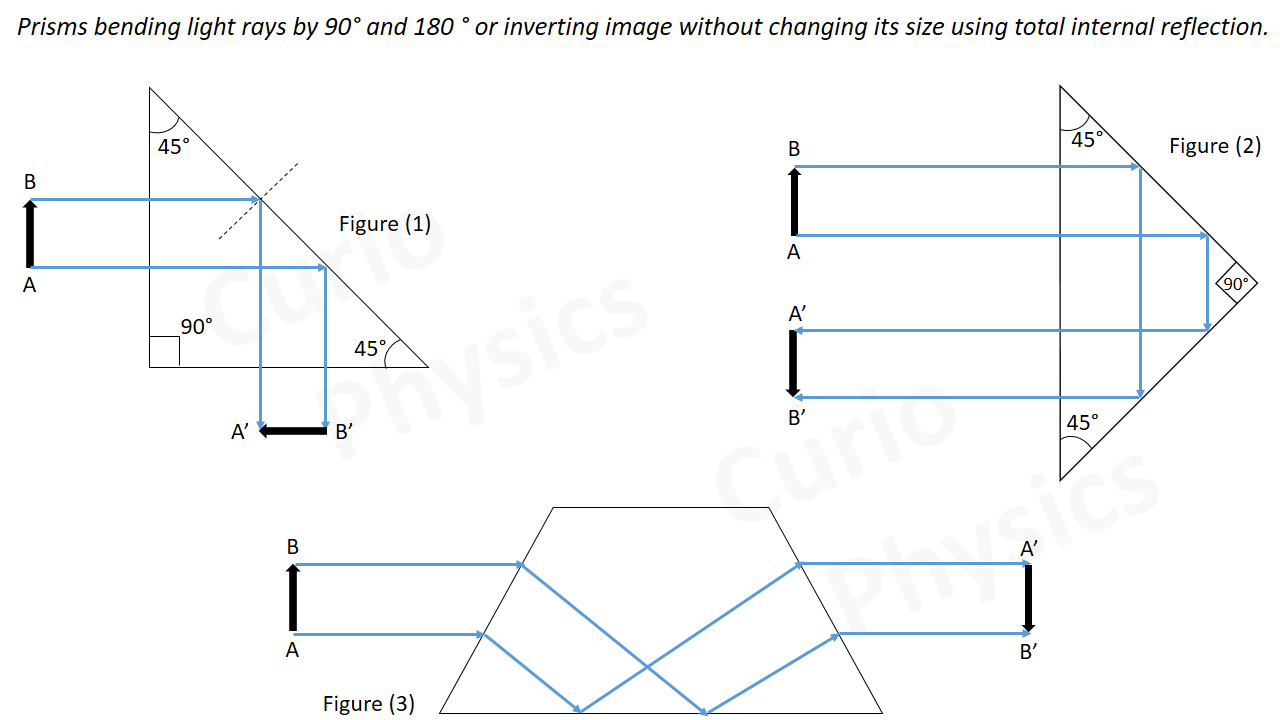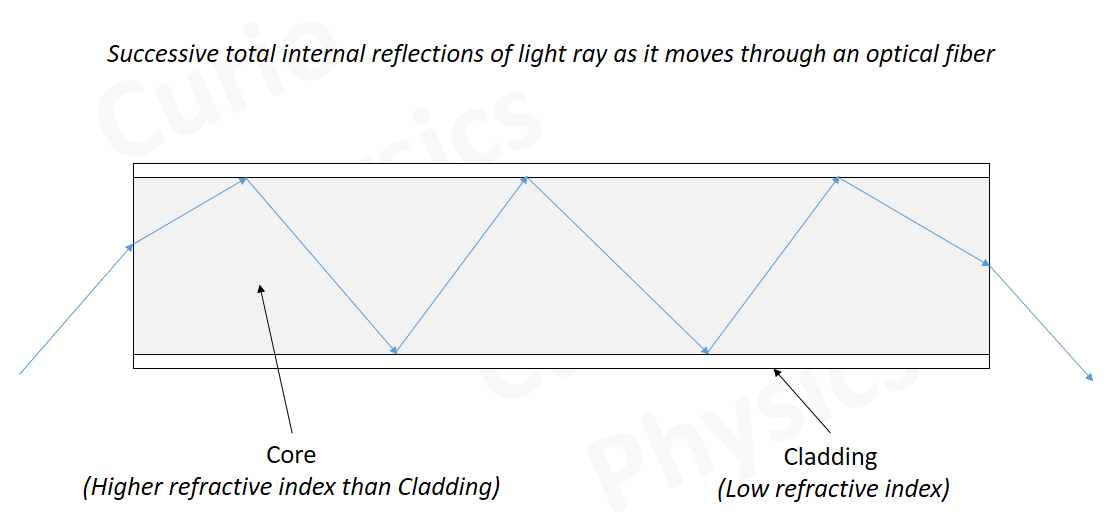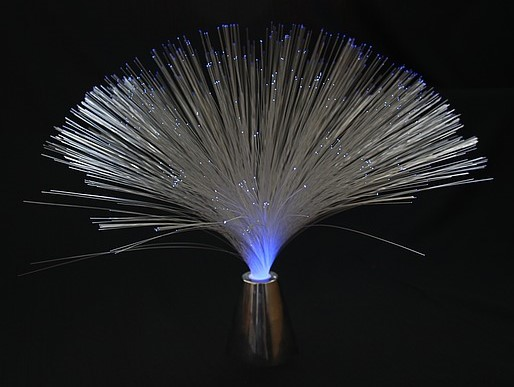# Total Internal Reflection

##### What is Total Internal Reflection?

When light travels from an optically denser medium to a rarer medium at the interface, it is partly reflected back into the same medium and partly refracted to the second medium. This reflection is called the internal reflection.

We know that when a ray of light enters from a denser medium to a rarer medium, it bends away from the normal (Refraction of light) , consider the figure given below :-The ray AO1 in above figure, is partially refracted(transmitted) along O1B and partially reflected along O1C, the angle of refraction (r1) being larger than the angle of incidence (i1) because the light ray travels from denser to rarer medium.

Now consider the ray AO2, here the angle of incidence(i2) is increased, so the angle of refraction(r2) also increases. In this we if we go on increasing the angle of incidence, the angle of refraction also increases and for the ray AO3, the angle of refraction becomes 90°, i.e., the refracted ray grazes along the air-water interface(Ray AO3D in figure).

Critical Angle (iC) :- The angle of incidence in the denser medium, for which the refracted ray grazes along the interface (i.e., the the angle of refraction is  90º), is called the critical angle (iC) for the given pair of media.

Now if the angle of incidence is increased still further (e.g., the ray AO4), refraction is not possible, and the incident ray is totally reflected. This is called total internal reflection.

##### Definition of total internal reflection

If a ray of light travelling from denser to rarer medium strikes the boundary at an angle greater than the critical angle(iC), then the light ray is totally reflected in the denser medium and no part of the light ray is refracted, this is called total internal reflection.

##### Why this is called total internal reflection ?

When light gets reflected by a surface, normally some fraction of it gets absorbed by the reflecting surface. The reflected ray, therefore, is always less intense than the incident ray, howsoever smooth the reflecting surface may be. But on the other hand, in total internal reflection, no transmission of light takes place and light ray is 100% reflected back in the denser medium. This is the reason this phenomenon is called total (100%) internal reflection.

##### Snell’s law and Total Internal Reflection

As we can see from the diagram, that as the angle of incidence(i) increases, the angle of refraction(r) also increases and at point of incidence O3, the value of angle of incidence is maximum(critical angle iC). For angle of incidence i > iC, Snell’s Law is not obeyed.

So we can also define Critical Angle as “the maximum value of angle of incidence in the denser medium, for which Snell’s Law is obeyed for the given pair of media.”

Applying Snell’s Law at point O3, we get$\displaystyle \frac{Sin{{i}_{C}}}{Sin90{}^\circ }=\frac{{{n}_{air}}}{{{n}_{water}}}=\frac{1}{{{n}_{denser}}}$     …..(1)

Here ndenser is the refractive index of water(or more accurately the refractive index of denser medium).

From above equation we get,$\displaystyle Sin{{i}_{C}}=\frac{1}{{{n}_{denser}}}$     …..(2)

The refractive index of denser medium with respect to rarer medium will be$\displaystyle {{n}_{denser}}=\frac{1}{Sin{{i}_{C}}}$     …..(3)

From equation (3), if we know the critical angle for a given pair of media, then we can find the refractive index of denser medium with respect to rarer medium.

##### Conditions for Total Internal Reflection

Following are the two conditions for the total internal reflection to take place:

• The ray of light must travel from a denser medium into a rarer medium.
• The angle of incidence in the denser medium must be greater than the critical angle for that pair of media.

##### Examples of Total Internal Reflection

(i) Mirage :- We are able to see an object, when light rays after striking the object, reach our eyes. Normally light rays travel in a straight line, and the object is seen at its actual position.

When the light rays of Sun strikes the surface of Earth, then first the layers of air near the surface of earth are heated. As a result on hot summer days, the air near the ground becomes hotter than the air at higher levels. The density of air affects its the refractive index, as the air becomes hotter, its refractive index decreases. Hotter air is less dense, and has smaller refractive index than the cooler air. If the air currents are small, that is, the air is still, the optical density at different layers of air increases with height.

Now if an observer see a tall object such as a tree, the light rays passes through the layers of air whose refractive index decreases towards the ground. Thus, a ray of light from such an object successively bends away from the normal and a situation arises where angle of incidence becomes greater than critical angle then the light rays undergoes total internal reflection. This is shown in figure below :-Now to an observer, the light appears to be coming from somewhere below the ground. It creates an illusion in the mind of observer that light is being reflected from the ground, say, by a pool of water near the tall object. This phenomenon of illusion that there is a pond of water is called mirage.

During a hot summer day, while moving in a bus or a car on a highway, sometimes we notice that there is a distant patch of road which appears to be wet. But, we do not find any evidence of wetness when you reach that spot. This is also due to mirage.

Also notice that is the day is not too hot, then the density of different layers of air remains almost same and so the refractive index. In that case we do not observe mirage, because the light rays coming from the object do not undergo total internal reflection and and travel in straight line so the object is seen by an observer at its place. This is shown in figure below :-(ii) Diamond :-  The critical angle for diamond-air interface is ≅ 24.4° which is very small. So once light enters a diamond, it is very likely to undergo total internal reflection inside it. Diamonds are cut in such a way, that multiple total internal reflections occurs inside which makes diamonds to sparkle so brilliantly.

(iii) Prism :- For some specific purposes, prisms are designed to bend light rays(using total internal reflection) by 90º[Figure(1)] or by 180º[Figure(2)] and in some cases to invert images without changing their size[Figure(3)].(iv) Optical fibers (or fibre in British English) :-

An optical fiber is a flexible, transparent fiber, slightly thicker than the diameter of a human hair, fabricated with high quality composite glass/quartz fiber used for transmitting audio and video signals through long distances at higher bandwidths (data transfer rates) than electrical cables.Working :- Optical fibers make use of the phenomenon of total internal reflection. The inner part of each fiber consists of a core and cladding. The refractive index of the material of the core is higher than that of the cladding and optical fibers are fabricated in such a way that light reflected at one side of inner surface strikes the other at an angle larger than the critical angle. Even if the fiber is bent, light can easily travel along its length.

When a signal in the form of light enters at one end of the fiber at a suitable angle, it undergoes repeated total internal reflections along the length of the fiber and finally comes out at the other end as shown in the figure below :-Uses of optical fiber :-

• For transmitting and receiving electrical signals (first signals are converted to light by suitable transducers).
• For transmission of optical signals.
• Used as a ‘light pipe’ for visual examination of internal organs
like esophagus, stomach and intestines.
• In decorative lamp as fine plastic fibers as shown in figure below :-A bundle of fibers is fixed over an electric lamp. When the lamp is switched on, the light travels from the bottom of each fiber and appears at the tip of its free end as a dot of light.

Next Topic :-    Spherical Refracting Surface